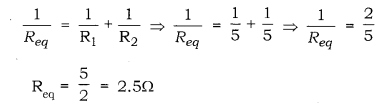# A wire of resistance 10 ohm is bent in the form of a closed circle

A wire of resistance 10 ohm is bent in the form of a closed circle. What is the effective resistance between the two points at the ends of any diameter of the circle?

As the wire is bent in the form of a closed circle, it will behave like two resistors of 5Ω each, connected in parallel. Therefore, the equivalent resistance can be calculated as:The effective resistance between the two points at the ends, of any diameter of the circle is 2.5Ω